Next: Generalization to the n-var Up: Graphical solution of 2-var Previous: Infeasible 2-var LP's

#### Unbounded 2-var LP's

In the LP's considered above, the feasible region (if not empty) was a bounded area of the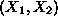-plane. For this kind of problems it is obvious that all values of the LP objective function (and therefore the optimal) are bounded. Consider however the following LP: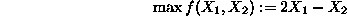s.t.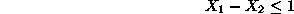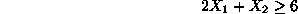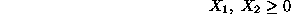The feasible region and the direction of improvement for the isoprofit lines for this problem are given in Figure 6.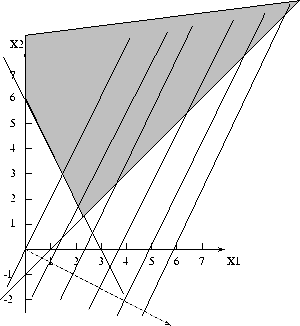Figure 6: An unbounded LP

It is easy to see that the feasible region of this problem is unbounded, and furthermore, the orientation of the isoprofit lines is such that no matter how far we ``slide'' these lines in the direction of increasing the objective function, they will always share some points with the feasible region. Therefore, this is an example of a (2-var) LP whose objective function can take arbitrarily large values. Such an LP is characterized as unbounded. Notice, however, that even though an unbounded feasible region is a necessary condition for an LP to be unbounded, it is not sufficient; to convince yourself, try to graphically identify the optimal solution for the above LP in the case that the objective function is changed to: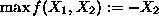.

Summarizing the above discussion, we have shown that a 2-var LP can either

• have a unique optimal solution which corresponds to a ``corner'' point of the feasible region, or
• have many optimal solutions that correspond to an entire ``edge'' of the feasible region, or
• be unbounded, or
• be infeasible.

In the next section, we generalize this geometrical description of the LP solution space for the n-var LP case, and we provide a brief (informal) derivation of the Fundamental Theorem of Linear Programming. The latter states that if an LP has a bounded optimal solution, then it must have one which is an extreme point of its feasible region. The Simplex algorithm to be discussed in the last section of this set of notes essentially exploits this fundamental result to reduce the space to be searched for an optimal solution.

Finally, you can actively try the graphical approach to the solution of 2-var LP's discussed above, by using the code developed by the group of Professors Ken Goldberg and Ilan Adler, at the University of Berkeley, Dept. of Industrial Engineering and Operations Research. Notice that the problem formulation uses a "minimization" objective, and all variables are considered to be "urs". Therefore, (i) any maximization problem must be turned into a minimization one (can you think of an easy way?), and (ii) all sign restrictions must be introduced in the formulation explicitly. Other than that, the software is self-explanatory.